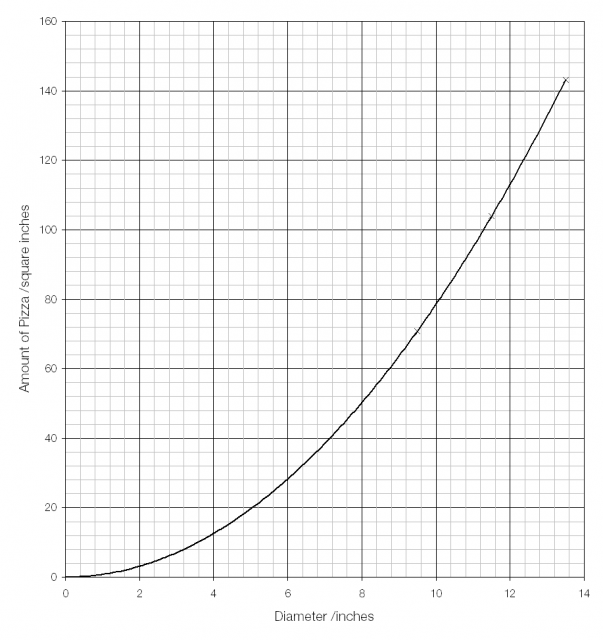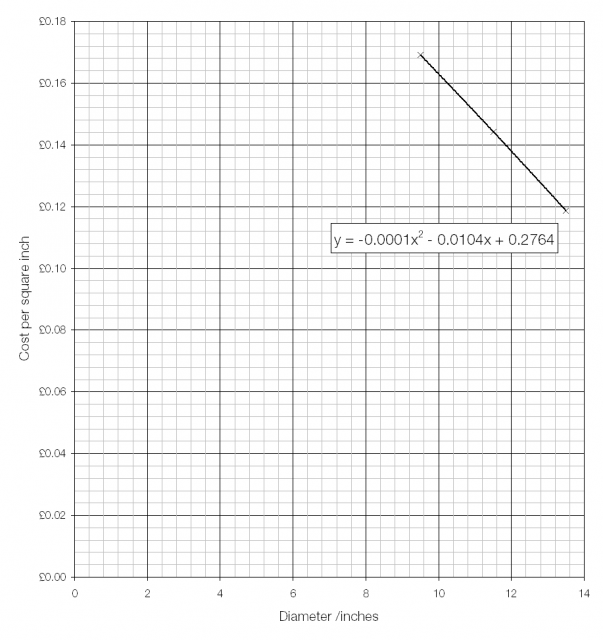# How big are pizzas?

Is a 12-inch pizza twice as big as a 6-inch pizza?

Simply put, no. The amount of pizza (its area) is proportional to the square of its diameter, so a 12-inch pizza is actually four times bigger than a 6-inch one.Looking at the cost per square inch shows an interesting pattern: as size increases, the cost per square inch goes down. (Data from Dominos Pizza.)If we take the trend that the data is following and solve the equation of the trendline (line of best fit) then we find two possible solutions: the cost per square inch falls to £0.00 at a diameter of either −128 inches or 22 inches. Since a pizza with a diameter of negative 128 inches is clearly ridiculous, it’s the free 22-inch pizza that is interesting.